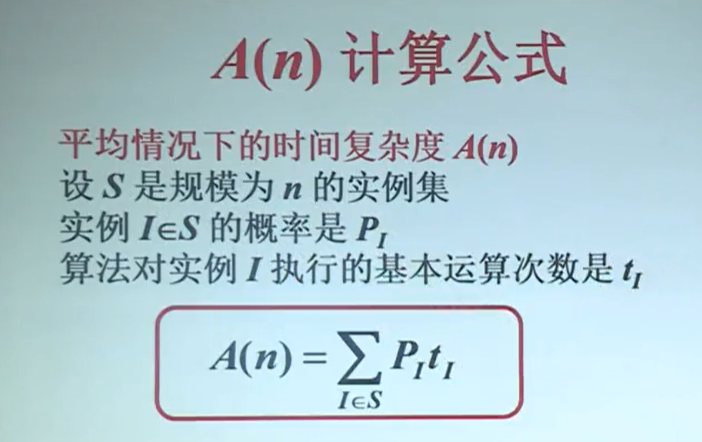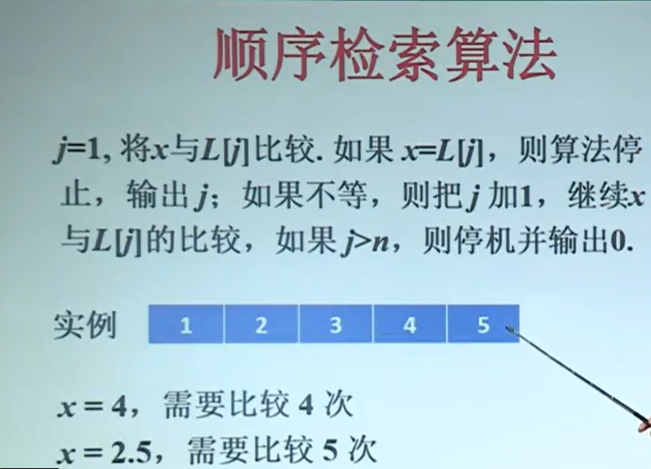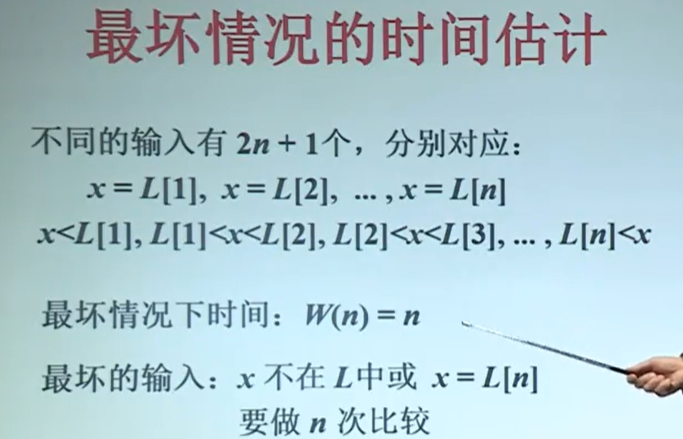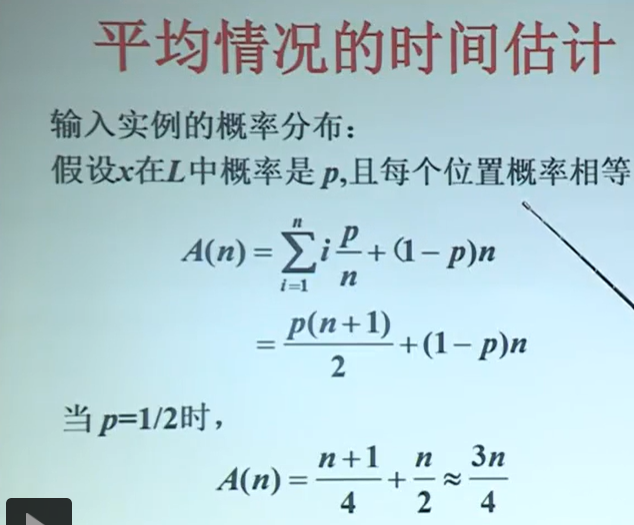• 时间复杂度计算(转载)   学习数据结构时，觉得时间复杂度计算很复杂，怎么也看不懂，差不多三年之后，还是不懂，马上就要找工作了，赶紧恶补一下吧： 首先了解一下几个概念。一个是时间复杂度，一个是...

时间复杂度计算(转载)

学习数据结构时，觉得时间复杂度计算很复杂，怎么也看不懂，差不多三年之后，还是不懂，马上就要找工作了，赶紧恶补一下吧： 首先了解一下几个概念。一个是时间复杂度，一个是渐近时间复杂度。前者是某个算法的时间耗费，它是该算法所求解问题规模n的函数，而后者是指当问题规模趋向无穷大时，该算法时间复杂度的数量级。 当我们评价一个算法的时间性能时，主要标准就是算法的渐近时间复杂度，因此，在算法分析时，往往对两者不予区分，经常是将渐近时间复杂度T(n)=O(f(n))简称为时间复杂度，其中的f(n)一般是算法中频度最大的语句频度。 此外，算法中语句的频度不仅与问题规模有关，还与输入实例中各元素的取值相关。但是我们总是考虑在最坏的情况下的时间复杂度。以保证算法的运行时间不会比它更长。 常见的时间复杂度，按数量级递增排列依次为：常数阶O(1)、对数阶O(log2n)、线性阶O(n)、线性对数阶O(nlog2n)、平方阶O(n^2)、立方阶O(n^3)、k次方阶O(n^k)、指数阶O(2^n)。 1. 大O表示法 定义  设一个程序的时间复杂度用一个函数 T(n) 来表示，对于一个查找算法，如下：   int seqsearch( int a[], const int n, const int x)  {  int i = 0;  for (; a[i] != x && i < n ; i++ );  if ( i == n) return -1;  else return i;  }  这个程序是将输入的数值顺序地与数组中地元素逐个比较，找出与之相等地元素。   在第一个元素就找到需要比较一次，在第二个元素找到需要比较2次，……　，在第n个元素找到需要比较n次。对于有n个元素的数组，如果每个元素被找到的概率相等，那么查找成功的平均比较次数为:   f(n) = 1/n (n + (n-1) + (n-2) + ... + 1) = (n+1)/2 = O(n)   这就是传说中的大O函数的原始定义。 用大O来表述  要全面分析一个算法，需要考虑算法在最坏和最好的情况下的时间代价，和在平均情况下的时间代价。对于最坏情况，采用大O表示法的一般提法（注意，这里用的是“一般提法”）是：当且仅当存在正整数c和n0,使得 T(n) <= c*f(n)对于所有的n >= n0 都成立。则称该算法的渐进时间复杂度为T(n) = O(f(n))。这个应该是高等数学里面的第一章极限里面的知识。这里f(n) = (n+1)/2, 那么c * f(n)也就是一个一次函数。就是在图象上看就是如果这个函数在c*f(n)的下面，就是复杂度为T(n) = O(f(n))。 对于对数级，我们用大O记法记为O(log2N)就可以了。  规则 1） 加法规则  T(n,m) = T1(n) + T2(n) = O ( max (f(n), g(m) )  2) 乘法规则  T(n,m) = T1(n) * T2(m) = O (f(n) * g(m))  3)一个特例  在大O表示法里面有一个特例，如果T1(n) ＝ O©， c是一个与n无关的任意常数，T2(n) = O ( f(n) ) 则有  T(n) = T1(n) * T2(n) = O ( c*f(n) ) = O( f(n) ).   也就是说，在大O表示法中，任何非0正常数都属于同一数量级，记为O(1)。  4)一个经验规则  有如下复杂度关系  c < log2N < n < n * Log2N < n^2 < n^3 < 2^n < 3^n < n!  其中c是一个常量，如果一个算法的复杂度为c 、 log2N 、n 、 n*log2N ,那么这个算法时间效率比较高 ，如果是 2^n , 3^n ,n!,那么稍微大一些的n就会令这个算法不能动了，居于中间的几个则差强人意. 1）基本知识点：没有循环的一段程序的复杂度是常数，一层循环的复杂度是O(n),两层循环的复杂度是O(n^2)? （我用^2表示平方，同理 ^3表示立方）； 2）二维矩阵的标准差，残差，信息熵，fft2,dwt2,dct2的时间复杂度: 标准差和残差可能O(n)，FFT2是O(nlog(n))，DWT2可能也是O(nlog(n))；信息熵要求概率，而dct的过程和jpeg一样。因为和jpeg一样,对二难矩阵处理了.Y=T*X*T'，Z=Y.*Mask，这样子,还有分成8*8子图像了; 3)example： 1、设三个函数f,g,h分别为 f(n)=100n^3+n^2+1000 , g(n)=25n^3+5000n^2 , h(n)=n^1.5+5000nlgn 请判断下列关系是否成立： （1） f(n)=O(g(n)) （2） g(n)=O(f(n)) （3） h(n)=O(n^1.5) （4） h(n)=O(nlgn) 这里我们复习一下渐近时间复杂度的表示法T(n)=O(f(n))，这里的"O"是数学符号，它的严格定义是"若T(n)和f(n)是定义在正整数集合上的两个函数，则T(n)=O(f(n))表示存在正的常数C和n0 ,使得当n≥n0时都满足0≤T(n)≤C?f(n)。"用容易理解的话说就是这两个函数当整型自变量n趋向于无穷大时，两者的比值是一个不等于0的常数。这么一来，就好计算了吧。 ◆ (1)成立。题中由于两个函数的最高次项都是n^3,因此当n→∞时，两个函数的比值是一个常数，所以这个关系式是成立的。 ◆ （2）成立。与上同理。 ◆ （3）成立。与上同理。 ◆ （4）不成立。由于当n→∞时n^1.5比nlgn递增的快，所以h(n)与nlgn的比值不是常数，故不成立。 2、设n为正整数，利用大"O"记号，将下列程序段的执行时间表示为n的函数。 (1) i=1; k=0 while(i<n) { k=k+10*i;i++; } 解答：T(n)=n-1， T(n)=O(n)， 这个函数是按线性阶递增的。 (2) x=n; // n>1 while (x>=(y+1)*(y+1)) y++; 解答：T(n)=n1/2 ，T(n)=O(n1/2)，最坏的情况是y=0，那么循环的次数是n1/2次，这是一个按平方根阶递增的函数。 (3) x=91; y=100; while(y>0) if(x>100) {x=x-10;y--;} else x++; 解答： T(n)=O(1)，这个程序看起来有点吓人，总共循环运行了1000次，但是我们看到n没有? 没。这段程序的运行是和n无关的，就算它再循环一万年，我们也不管他，只是一个常数阶的函数。 同一问题可用不同算法解决，而一个算法的质量优劣将影响到算法乃至程序的效率。算法分析的目的在于选择合适算法和改进算法。一个算法的评价主要从时间复杂度和空间复杂度来考虑。   1、时间复杂度  （1）时间频度 一个算法执行所耗费的时间，从理论上是不能算出来的，必须上机运行测试才能知道。但我们不可能也没有必要对每个算法都上机测试，只需知道哪个算法花费的时间多，哪个算法花费的时间少就可以了。并且一个算法花费的时间与算法中语句的执行次数成正比例，哪个算法中语句执行次数多，它花费时间就多。一个算法中的语句执行次数称为语句频度或时间频度。记为T(n)。  （2）时间复杂度 在刚才提到的时间频度中，n称为问题的规模，当n不断变化时，时间频度T(n)也会不断变化。但有时我们想知道它变化时呈现什么规律。为此，我们引入时间复杂度概念。  一般情况下，算法中基本操作重复执行的次数是问题规模n的某个函数，用T(n)表示，若有某个辅助函数f(n),使得当n趋近于无穷大时，T（n)/f(n)的极限值为不等于零的常数，则称f(n)是T(n)的同数量级函数。记作T(n)=Ｏ(f(n)),称Ｏ(f(n)) 为算法的渐进时间复杂度，简称时间复杂度。  在各种不同算法中，若算法中语句执行次数为一个常数，则时间复杂度为O(1),另外，在时间频度不相同时，时间复杂度有可能相同，如T(n)=n2+3n+4与T(n)=4n2+2n+1它们的频度不同，但时间复杂度相同，都为O(n2)。  按数量级递增排列，常见的时间复杂度有： 常数阶O(1),对数阶O(log2n),线性阶O(n), 线性对数阶O(nlog2n),平方阶O(n2)，立方阶O(n3),...， k次方阶O(nk),指数阶O(2n)。随着问题规模n的不断增大，上述时间复杂度不断增大，算法的执行效率越低。  2、空间复杂度  与时间复杂度类似，空间复杂度是指算法在计算机内执行时所需存储空间的度量。记作: S(n)=O(f(n))  我们一般所讨论的是除正常占用内存开销外的辅助存储单元规模。讨论方法与时间复杂度类似，不再赘述。
（3）渐进时间复杂度评价算法时间性能 　　主要用算法时间复杂度的数量级(即算法的渐近时间复杂度)评价一个算法的时间性能。 【例3．7】有两个算法A1和A2求解同一问题，时间复杂度分别是T1(n)=100n2，T2(n)=5n3。 （1）当输入量n＜20时，有T1(n)＞T2(n)，后者花费的时间较少。 （2）随着问题规模n的增大，两个算法的时间开销之比5n3/100n2=n/20亦随着增大。即当问题规模较大时，算法A1比算法A2要有效地多。 它们的渐近时间复杂度O(n2)和O(n3)从宏观上评价了这两个算法在时间方面的质量。在算法分析时，往往对算法的时间复杂度和渐近时间复杂度不予区分，而经常是将渐近时间复杂度T(n)=O(f(n))简称为时间复杂度，其中的f(n)一般是算法中频度最大的语句频度。 【例3．8】算法MatrixMultiply的时间复杂度一般为T(n)=O(n3)，f(n)=n3是该算法中语句(5)的频度。下面再举例说明如何求算法的时间复杂度。  【例3．9】交换i和j的内容。       Temp=i;       i=j;       j=temp;  　　以上三条单个语句的频度均为1，该程序段的执行时间是一个与问题规模n无关的常数。算法的时间复杂度为常数阶，记作T(n)=O(1)。       如果算法的执行时间不随着问题规模n的增加而增长，即使算法中有上千条语句，其执行时间也不过是一个较大的常数。此类算法的时间复杂度是O(1)。  【例3．10】变量计数之一。 (1) x=0;y=0; (2) for(k-1;k<=n;k++) (3)     x++; (4) for(i=1;i<=n;i++) (5)       for(j=1;j<=n;j++) (6)         y++;  　　一般情况下，对步进循环语句只需考虑循环体中语句的执行次数，忽略该语句中步长加1、终值判别、控制转移等成分。因此，以上程序段中频度最大的语句是(6)，其频度为f(n)=n2，所以该程序段的时间复杂度为T(n)=O(n2)。 　　当有若干个循环语句时，算法的时间复杂度是由嵌套层数最多的循环语句中最内层语句的频度f(n)决定的。  【例3．11】变量计数之二。 (1) x=1; (2) for(i=1;i<=n;i++) (3)       for(j=1;j<=i;j++) (4)           for(k=1;k<=j;k++) (5)               x++;  　　该程序段中频度最大的语句是(5)，内循环的执行次数虽然与问题规模n没有直接关系，但是却与外层循环的变量取值有关，而最外层循环的次数直接与n有关，因此可以从内层循环向外层分析语句(5)的执行次数：  则该程序段的时间复杂度为T(n)=O(n3/6+低次项)=O(n3)。  （4）算法的时间复杂度不仅仅依赖于问题的规模，还与输入实例的初始状态有关。 【例3．12】在数值A[0..n-1]中查找给定值K的算法大致如下：    (1)i=n-1;           (2)while(i>=0&&(A[i]!=k))       (3)     i--;       (4)return i;         此算法中的语句(3)的频度不仅与问题规模n有关，还与输入实例中A的各元素取值及K的取值有关: ①若A中没有与K相等的元素，则语句(3)的频度f(n)=n； ②若A的最后一个元素等于K,则语句(3)的频度f(n)是常数0。  （5）最坏时间复杂度和平均时间复杂度 　　最坏情况下的时间复杂度称最坏时间复杂度。一般不特别说明，讨论的时间复杂度均是最坏情况下的时间复杂度。       这样做的原因是：最坏情况下的时间复杂度是算法在任何输入实例上运行时间的上界，这就保证了算法的运行时间不会比任何更长。 【例3．19】查找算法【例1·8】在最坏情况下的时间复杂度为T(n)=0(n)，它表示对于任何输入实例,该算法的运行时间不可能大于0(n)。       平均时间复杂度是指所有可能的输入实例均以等概率出现的情况下，算法的期望运行时间。       常见的时间复杂度按数量级递增排列依次为：常数0(1)、对数阶0(log2n)、线形阶0(n)、线形对数阶0(nlog2n)、平方阶0(n2)立方阶0(n3)、…、k次方阶0(nk)、指数阶0(2n)。显然，时间复杂度为指数阶0(2n)的算法效率极低，当n值稍大时就无法应用。       类似于时间复杂度的讨论，一个算法的空间复杂度(Space Complexity)S(n)定义为该算法所耗费的存储空间，它也是问题规模n的函数。渐近空间复杂度也常常简称为空间复杂度。算法的时间复杂度和空间复杂度合称为算法的复杂度。


展开全文算法 C
• 主要介绍了科学知识：时间复杂度计算方法,本文介绍了问题的定义、时间复杂度计算步骤、时间复杂度计算规则等内容,需要的朋友可以参考下
• 算法时间复杂度计算
算法时间复杂度计算
定义：
一般情况下，算法时间复杂度就是算法中基本操作重复执行的次数。
计算方法：
先找出算法的基本操作，然后计算出它们的执行次数，忽略常量、低次幂和高次幂的系数。用大O来表示时间复杂度。
常见时间复杂度：
1.常数时间复杂度O(1)：如果算法的执行时间不随着问题规模n的增加而增长，即使算法中有上千条语句，其执行时间也不过是一个较大的常数。此类算法的时间复杂度是O(1)。
2.对数阶O(log2n)、线性对数阶O(nlog2n)，除了常数阶以外，该种效率最高。
3.O(z^2)：指数阶时间复杂度，该种不实用，指数爆炸性。
4.对数阶log2n（以2为底10的对数）与lg(n)是等价的，因为对数换底公式：log(a,b)=log(c,b)/log(c,a)  =》 忽略掉系数，二者等价。
5.含极限时间复杂度： 1/n 当n趋于无穷大时，1/n趋向于0，所以2*(1/n)*(1/log2n) + 4*(1/log2n) + 3=0。
复杂度与时间效率的关系：
c < log2n < n < n*log2n < n2 < n3 < 2n < 3n < n! （c是一个常量） |--------------------------|--------------------------|-------------|            较好                           一般                      较差
一个例子
int num1, num2;for(int i=0; i<n; i++){  num1 += 1;  for(int j=1; j<=n; j*=2){   num2 += num1;  }
}

基本语句：两重for循环中的语句。
第一重for循环的时间复杂度为n。
对于第二重循环的时间复杂度为：
j每循环一次乘了2。
J初始为1，所以循环x从之后j=2^x
J>n时停止循环，也就是2^x  > n，此时x=log2n。
所以j循环了log2n次。
所以整个程序的时间复杂度为nlog2n。
参考地址
http://blog.csdn.net/firefly_2002/article/details/8008987
http://www.zhihu.com/question/20503898
http://univasity.iteye.com/blog/1164707

展开全文算法
• 慕课学习-平均时间复杂度计算 参照A(n)前半部分公式，可得在1-p的情况下： [(1-p)/n*n1]2*n3 需要比较n次 ↩︎ 每一次的结果，等同于 前半部分的i*p/n ↩︎ 总共有n个相同的"2" ↩︎ ...
慕课学习-平均时间复杂度计算参照A(n)前半部分公式，可得在1-p的情况下： [(1-p)/n*n1]2*n3

需要比较n次 ↩︎ 每一次的结果，等同于 前半部分的i*p/n ↩︎ 总共有n个相同的"2" ↩︎

展开全文• 递归算法的时间复杂度计算 递归时间复杂度的计算本质在于 递归次数*每次递归中的操作数 利用二叉树进行递归调用：O(logn),每次递归调用都是n/2。
递归算法的时间复杂度计算
递归时间复杂度的计算本质在于递归次数*每次递归中的操作数
利用二叉树进行递归调用：O(logn),每次递归调用都是n/2。
展开全文java
• 大小514,271 分治法与时间复杂度计算.pdf，希望对大家有帮助。
• 实验一 算法的时间复杂度 实验目的与要求 熟悉C/C++语言的集成开发环境 通过本实验加深对算法分析基础知识的理解 软件环境 操作系统windows7 旗舰版 集成开发环境 visual studio 2010 旗舰版 硬件环境 处理器因特尔 ...
• 黄色标亮-划重点背诵+自己的理解，绿色标...二分法查找 的 时间复杂度计算 设 查找数据的长度为n，每次查找后数据长度减半，则有 查找次数 数据长度 第1次查找 n/2 ……   第k次查找 n/2^k 最坏情况数据结构 学习笔记
• 斐波那契数列——递归法时间复杂度计算 画图：以F（6）为例：斐波那契数列到第六个数停止 每个节点运行都会开辟空间，使用时间 为了方便计算，把第五层的f（2）和f（1）放在第四层最右边，不会影响时间复杂度计算 ...
• 本问题源于《算法设计分析》，仔细并分析了阶乘问题时间复杂度计算。采用递推方法求解
• 高斯消元法的时间复杂度计算1、时间复杂度定义2、几种常见复杂度执行效率的比较3、时间复杂度的计算4、高斯消元法的时间复杂度     算法的时间复杂度通常用来反映程序执行时间随输入规模增长而增长的量级，在很大...算法
• 数据结构的核心思想是通过数据结构的思维来优化代码的算法，以此来提升程序的执行性能，缩短程序执行的时间。下面我来举两个例子，以此来说明数据结构的时间复杂度计算问题。数据结构 线性表 顺序表
• 时间复杂度计算 此笔记来源于左神算法，只用做笔记使用，已注明来源。 此事件复杂度只适用于递归算法，并且递归过程中要求数据规模大体相同 T(N)=a∗T(Nb)+O(Nd)T(N)=a*T\left(\frac{N}{b}\right)+O(N^d)T(N)=a∗T...算法
• 4.嵌套循环时间复杂度计算翻译自: https://hackernoon.com/how-we-reduced-the-time-complexity-from-18-days-to-4-5-minutes-a4bdfa72e5234.嵌套循环时间复杂度计算java python leetcode 数据结构 机器学习
• 时间复杂度计算 for (i = 0; i < n; i++) { for (j = 0; j < n; j++) { cout << "csdn" << endl; } } for (i = 0; i < n; i++) { for (j = i; j < n; j++)数据结构
• 时间复杂度计算主要分为3个步骤： 先找出算法的基本操作 计算基本语句的执行次数的数量级 用O记号表示算法的时间性能 2. 求两数最大公约数 问题描述：求a, b两正整数的公约数。 相关定理：已知a,b,c为正整数，若a...最大公约数
• 线性表——顺序表——时间复杂度计算    在之前的文章中的示例1和示例2中，我们通过观察可以发现，当在顺序存储结构的线性表中某个位置上插入或删除一个数据元素时，其时间主要耗费在移动元素上（换句话说，移动...线性表 顺序表
• 时间复杂度公示： 下面分析一段代码： 2、3、4行每一行都需要1个单位的执行时间，5、6行循环了N边，需要2N个单位时间，7、8行执行了n^2遍，所以一共需要执行时间：（2N ^2 + 2N + 3 ）*单位时间。 我们用T(n) 表示...
• 首先，我们需要知道的是 时间复杂度计算的是一个程序大致执行了多少个语句（之前我认为是要计算到底执行了多少秒，是我天真了，怎么可能了），时间复杂度计算允许省去一些影响小的因素（比如说后面会提到的n对n2...算法 java 数据结构
• ## java时间复杂度计算

千次阅读 2019-09-03 22:19:29
时间复杂度是指算法执行语句执行的次数。 常见的时间复杂度有以下几种： 描述 时间复杂度 常数阶 O(1) 对数阶 O(logn) 线性阶 O(n) 线性对数阶 O(nlogn) 平方阶 O(n²) 立方阶 O......